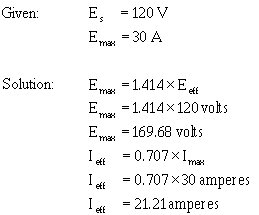Effective Value of a Sine Wave resistance, heat is produced. ">Custom SearchEFFECTIVE VALUE OF A SINE WAVE Emax, Eavg, I max, and Iavg are values used in ac measurements. Another value used is the EFFECTIVE value of ac This is the value of alternating voltage or current that will have the same effect on a resistance as a comparable value of direct voltage or current will have on the same resistance. In an earlier discussion you were told that when current flows in a resistance, heat is produced. When direct current flows in a resistance, the amount of electrical power converted into heat equals I2R watts. However, since an alternating current having a maximum value of 1 ampere does not maintain a constant value, the alternating current will not produce as much heat in the resistance as will a direct current of 1 ampere. Figure 1-15 compares the heating effect of 1 ampere of dc to the heating effect of 1 ampere of ac. Figure 1-15. - Heating effect of ac and dc.Examine views A and B of figure 1-15 and notice that the heat (70.7° C) produced by 1 ampere of alternating current (that is, an ac with a maximum value of 1 ampere) is only 70.7 percent of the heat (100° C) produced by 1 ampere of direct current. Mathematically,Therefore, for effective value of ac (I eff) = 0.707 X Imax. The rate at which heat is produced in a resistance forms a convenient basis for establishing an effective value of alternating current, and is known as the "heating effect" method. An alternating current is said to have an effective value of one ampere when it produces heat in a given resistance at the same rate as does one ampere of direct current. You can compute the effective value of a sine wave of current to a fair degree of accuracy by taking equally-spaced instantaneous values of current along the curve and extracting the square root of the average of the sum of the squared values. For this reason, the effective value is often called the "root-mean-square" (rms) value. Thus,Stated another way, the effective or rms value (Ieff) of a sine wave of current is 0.707 times the maximum value of current (Imax). Thus, I eff = 0.707 X Imax. When I eff is known, you can find Imax by using the formula Imax = 1.414 X Ieff. You might wonder where the constant 1.414 comes from. To find out, examine figure 1-15 again and read the following explanation. Assume that the dc in figure 1-15(A) is maintained at 1 ampere and the resistor temperature at 100°C. Also assume that the ac in figure 1-15(B) is increased until the temperature of the resistor is 100° C. At this point it is found that a maximum ac value of 1.414 amperes is required in order to have the same heating effect as direct current. Therefore, in the ac circuit the maximum current required is 1.414 times the effective current. It is important for you to remember the above relationship and that the effective value (I eff) of any sine wave of current is always 0.707 times the maximum value (Imax). Since alternating current is caused by an alternating voltage, the ratio of the effective value of voltage to the maximum value of voltage is the same as the ratio of the effective value of current to the maximum value of current. Stated another way, the effective or rms value (E eff) of a sine-wave of voltage is 0.707 times the maximum value of voltage (Emax),When an alternating current or voltage value is specified in a book or on a diagram, the value is an effective value unless there is a definite statement to the contrary. Remember that all meters, unless marked to the contrary, are calibrated to indicate effective values of current and voltage. Problem: A circuit is known to have an alternating voltage of 120 volts and a peak or maximum current of 30 amperes. What are the peak voltage and effective current values?Figure 1-16 shows the relationship between the various values used to indicate sine-wave amplitude. Review the values in the figure to ensure you understand what each value indicates. Figure 1-16. - Various values used to indicate sine-wave amplitude.Q.32 What is the most convenient basis for comparing alternating and direct voltages and currents? Q.33 What value of ac is used as a comparison to dc? Q.34 What is the formula for finding the effective value of an alternating current? Q.35 If the peak value of a sine wave is 1,000 volts, what is the effective (Eeff) value? Q.36 If Ieff = 4.25 ampere, what is Imax ?Integrated Publishing, Inc. - A (SDVOSB) Service Disabled Veteran Owned Small Business